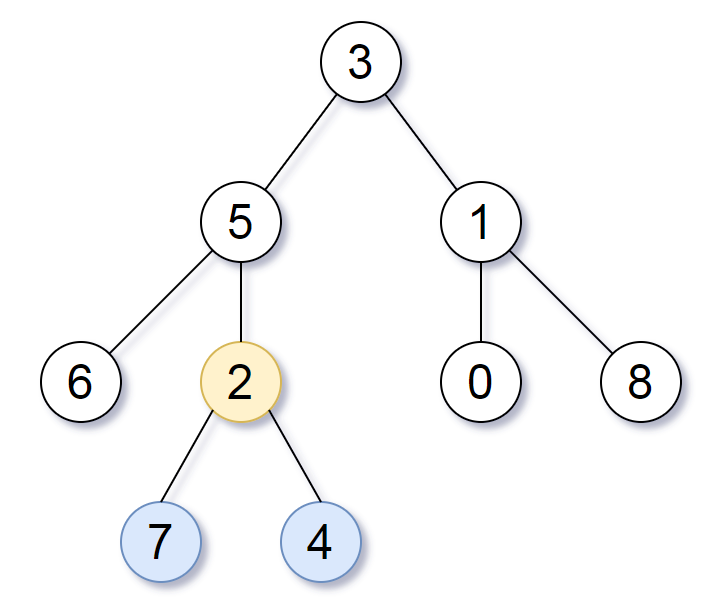Formatted question description: https://leetcode.ca/all/865.html

# 865. Smallest Subtree with all the Deepest Nodes (Medium)

Given the root of a binary tree, the depth of each node is the shortest distance to the root.

Return the smallest subtree such that it contains all the deepest nodes in the original tree.

A node is called the deepest if it has the largest depth possible among any node in the entire tree.

The subtree of a node is tree consisting of that node, plus the set of all descendants of that node.

Note: This question is the same as 1123: https://leetcode.com/problems/lowest-common-ancestor-of-deepest-leaves/

Example 1:Input: root = [3,5,1,6,2,0,8,null,null,7,4]
Output: [2,7,4]
Explanation: We return the node with value 2, colored in yellow in the diagram.
The nodes coloured in blue are the deepest nodes of the tree.
Notice that nodes 5, 3 and 2 contain the deepest nodes in the tree but node 2 is the smallest subtree among them, so we return it.


Example 2:

Input: root = 
Output: 
Explanation: The root is the deepest node in the tree.


Example 3:

Input: root = [0,1,3,null,2]
Output: 
Explanation: The deepest node in the tree is 2, the valid subtrees are the subtrees of nodes 2, 1 and 0 but the subtree of node 2 is the smallest.


Constraints:

• The number of nodes in the tree will be in the range [1, 500].
• 0 <= Node.val <= 500
• The values of the nodes in the tree are unique.

Related Topics:
Tree, Depth-first Search, Breadth-first Search, Recursion

## Solution 1.

## Solution 1.

cpp
// OJ: https://leetcode.com/problems/smallest-subtree-with-all-the-deepest-nodes/

// Time: O(N)
// Space: O(H)
class Solution {
int maxDepth = -1, target = 0, cnt = 0;
void count(TreeNode *root, int d) {
if (!root) return;
if (d > maxDepth) {
target = 1;
maxDepth = d;
} else if (d == maxDepth) ++target;
count(root->left, d + 1);
count(root->right, d + 1);
}
TreeNode *find(TreeNode *root, int d) {
if (!root) return NULL;
int before = cnt;
auto left = find(root->left, d + 1);
if (left) return left;
auto right = find(root->right, d + 1);
if (right) return right;
if (d == maxDepth) ++cnt;
return before == 0 && cnt == target ? root : NULL;
}
public:
TreeNode* lcaDeepestLeaves(TreeNode* root) {
count(root, 0);
return find(root, 0);
}
};


## Solution 2.

The lowest ancester is the highest node whose left and right subtrees have the same height.

// OJ: https://leetcode.com/problems/smallest-subtree-with-all-the-deepest-nodes/

// Time: O(N)
// Space: O(H)
class Solution {
pair<TreeNode*, int> dfs(TreeNode *root, int d = 0) { // latest node which has equal depth in left and right sub-trees; the corresponding height
if (!root) return {NULL, 0};
const auto &[left, ld] = dfs(root->left, d + 1);
const auto &[right, rd] = dfs(root->right, d + 1);
if (ld > rd) return {left, ld + 1};
else if (ld < rd) return{right, rd + 1};
return {root, ld + 1};
}
public:
TreeNode* lcaDeepestLeaves(TreeNode* root) {
return dfs(root).first;
}
};


Java

/**
* Definition for a binary tree node.
* public class TreeNode {
*     int val;
*     TreeNode left;
*     TreeNode right;
*     TreeNode(int x) { val = x; }
* }
*/
class Solution {
public TreeNode subtreeWithAllDeepest(TreeNode root) {
Map<Integer, List<TreeNode>> depthNodesMap = new HashMap<Integer, List<TreeNode>>();
Map<TreeNode, TreeNode> childParentMap = new HashMap<TreeNode, TreeNode>();
Queue<TreeNode> queue = new LinkedList<TreeNode>();
queue.offer(root);
int depth = 0;
while (!queue.isEmpty()) {
depth++;
List<TreeNode> list = new ArrayList<TreeNode>();
int size = queue.size();
for (int i = 0; i < size; i++) {
TreeNode node = queue.poll();
TreeNode left = node.left, right = node.right;
if (left != null) {
childParentMap.put(left, node);
queue.offer(left);
}
if (right != null) {
childParentMap.put(right, node);
queue.offer(right);
}
}
depthNodesMap.put(depth, list);
}
List<TreeNode> nodesList = depthNodesMap.get(depth);
if (nodesList == null)
return null;
while (nodesList.size() > 1) {
Set<TreeNode> set = new HashSet<TreeNode>();
int size = nodesList.size();
for (int i = 0; i < size; i++) {
TreeNode node = nodesList.remove(0);
TreeNode parent = childParentMap.get(node);
if (parent != null)
`